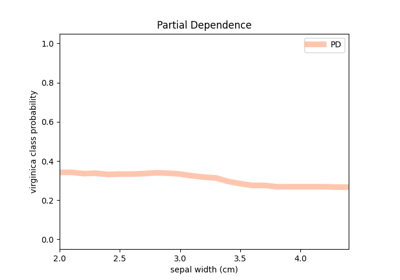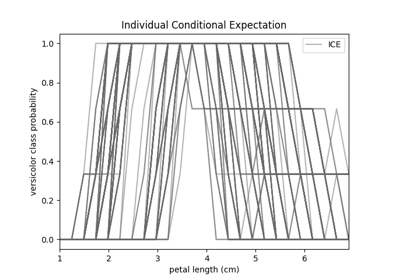# fatf.transparency.models.feature_influence.partial_dependence_ice¶

fatf.transparency.models.feature_influence.partial_dependence_ice(ice_array: numpy.ndarray, include_rows: Union[int, List[int], None] = None, exclude_rows: Union[int, List[int], None] = None) → numpy.ndarray[source]

Calculates Partial Dependence based on Individual Conditional Expectations.

Note

If you want to calculate Partial Dependence directly from a dataset and a classifier please see fatf.transparency.models.feature_influence.partial_dependence function.

Parameters
ice_arraynumpy.ndarray

Individual Conditional Expectation array for which Partial Dependence is desired.

include_rowsUnion[int, List[int]], optional (default=None)

Indices of rows that will be included in the PD calculation. If this parameter is specified, PD will only be calculated for the selected rows. If additionally exclude_rows is specified the selected rows will be a set difference between the two. This parameter can either be a list of indices or a single index (integer).

exclude_rowsUnion[int, List[int]], optional (default=None)

The indices of rows to be excluded from the PD calculation. If this parameter is specified and include_rows is not, these indices will be excluded from all of the rows. If both include and exclude parameters are specified, the rows included in the PD calculation will be a set difference of the two. This parameter can either be a list of indices or a single index (integer).

Returns
partial_dependence_arraynumpy.ndarray

A 2-dimensional array of (steps_number, n_classes) shape representing Partial Dependence for all of the classes for selected rows (data points).

Raises
IncorrectShapeError

The input array is not a 3-dimensional numpy array.

TypeError

Either include_rows or exclude_rows parameter is not None, an integer or a list of integers.

ValueError

The ice_array is not an unstructured numpy array or it is not a numerical array. One of the include_rows or exclude_rows indices is not valid for the input array.

## Examples using fatf.transparency.models.feature_influence.partial_dependence_ice¶